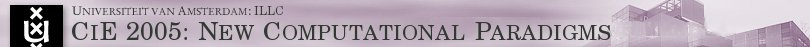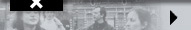CiE 2005: General InformationThe conference will cover many aspects of "Computability in Europe" (CiE), with a particular focus on New Computational Paradigms. These include prominently connections between computation and physical systems (e.g., quantum and analog computation, neural nets, molecular computation), but also covers new perspectives on models of computation arising from basic research in mathematical logic and theoretical computer science.

Computability Theory is central to large areas of theoretical computer science and mathematical logic. Traditionally, the computational model of the Turing machine (or mathematically equivalent models) has been used to reason about computation or computability. For general computability inquiries (with unbounded resources), the choice of the model of computation hardly matters; this often changes as soon as questions of efficiency or relative computation are investigated. In all areas of computability theory, alternative models of computation have been investigated, ranging from the most abstract (generalized recursion theory, infinite time Turing machines, computation relative to enumerations of data, mathematical models of emergence) to the very concrete (physical constructions of quantum computers, applications in neuroscience and learning theory, fine grained parallelism, swarm intelligence, neural nets, agents, games).

CiE 2005 is an interdisciplinary venue for researchers from computer science and mathematics focusing on New Computational Paradigms. These include prominently connections between computation and physical systems but also higher mathematical models of computation. The researchers from the different communities will exchange ideas, approaches and techniques in their respective work, thereby generating a wider community for work on computational issues that allows uniform approaches to diverse areas, the transformation of theoretical ideas into applicable projects, and general cross-fertilization transcending disciplinary borders.